# PRICE CATEGORY PREDICTION ON IMAGE CATEGORIES

1. PROBLEM OVERVIEW
2. DATASET SOURCE AND DESCRIPTION
4. APPROACH TO SOLVE THE PROBLEM
5. BASIC EXPLORATORY DATA ANALYSIS
6. CODE SNIPPETS AND OUTPUTS
7. FUTURE WORK
8. CODE FOR THE PROJECT
9. REFERENCES

# PROBLEM OVERVIEW:-

This problem which we would be dealing here is a multi-class classification problem of price range prediction for images of four categories. For each category, we would have to predict the price range of the particular object in the image.

# DATASET SOURCE AND DESCRIPTION:-

## SOURCE OF THE DATASET:-

The dataset for the problem can be found here.

## DATASET DESCRIPTION:-

The dataset consists of two folders named train and test respectively. These contain the following:-

1. train- There are four sub-folders named Cars, Perfumes, Real Estate and Watches. Each of these folders contain various images of objects belonging to the respective categories. There are a total of 1925 images belonging to the four categories.
2. test- There are four sub-folders named Cars, Perfumes, Real Estate and Watches. Each of these folders contain various images of objects belonging to the respective categories. There are a total of 404 images belonging to the four categories.
3. train.csv- A csv file specifying the name of the image file, it’s category of age in years, availability of special discount, price range and category to which this object belongs to.
4. test.csv- A csv file specifying the the name of the image file, it’s age in years, availability of special discount and category to which this object belongs to. The price range for the images along with the two categorical features of age and special discount availability has to be predicted.

The Key Performance Metric on which the algorithm has to be assessed or evaluated is 100*micro_averaged_f1_score.

We know that f1_score is the harmonic mean of precision and recall. Let’s deep dive into what are the different types of f1_score. There are mainly three types of f1-scores which are:-

1. Micro_averaged_f1_score- It is the harmonic mean of micro precision and micro recall. Micro Precision in case of a multi class classification is the same in terms of mathematical formula to precision i.e. TP/(TP + FP). In the case of Micro Precision for a multi-class classification, we calculate the False Positives(FP) as the number of incorrect predictions and True Positives as the total number of correct predictions for all the classes. For e.g:- A False Positive for class 1 is something which is predicted as class 1 but belongs to some other class originally. Micro Recall in case of a multi class classification is the same in terms of mathematical formula to recall i.e. TP/(TP + FN). We calculate the False Negatives (FN) also as the total number of incorrect predictions in case of a multi-class classification. For e.g:- A False Negative for class 1 is something which is predicted as some other class but originally belongs to class 1. In micro-averaged f1-score, micro precision = micro recall = micro f1-score = accuracy.
2. Macro_averaged_f1_score- It is the harmonic mean of macro precision and macro recall for each class and then at a total level, as simple average of the f1-scores of all the classes. The macro precision for class 1 is defined as TP/(TP + FP) where False Positives(FP) for class 1 is defined as the predictions done for class 1 which are actually belonging to some other classes. Similarly, the macro recall for class 1 is defined as TP/(TP + FN) where False Negatives(FN) for class 1 is defined as the predictions done for other classes but which actually belong to class 1. After we arrive at the macro precision and macro recall for each of the classes, we calculate the f1-score for each of the classes. Then we arrive at the macro-averaged f1-score by taking the average of the f1-score of all the classes.
3. Weighted f1_score- It is the weighted average of the f1-scores for each of the classes weighted by the number of samples present for each of the classes. So, say suppose we have 3 classes, a total of 100 data points and for each of the classes, we have 30, 40 and 30 data points, then the weights for each of the classes would be 30/100, 40/100 and 30/100. The weighted f1-score would be a sum of the products of the above weights and the f1-scores for each of the classes.

You can read more about f1-scores and their types in this blog.

# APPROACH TO SOLVE THE PROBLEM:-

We would take the following approach to solve the problem:-

1. Exploratory Data Analysis to uncover the class imbalance, correlation of each of the categorical features age and special discount availability with the price range and also checking the cdfs for the categorical feature age to verify whether this feature is a good feature for separating the price range for each of the categories.
2. Removing the features age and special discount availability from the analysis if they don’t make sense to the price ranges for each of the categories.
3. Training the pretrained VGG16 model by removing the top layer and adding some more Fully Connected Layers on the data to predict the various categories of images given by maximizing accuracy.
4. Visualizing the activations of the final fully connected layers in 2 dimensions for the categories and also for the price ranges.
5. Taking the activations of the final layer from the above trained model and then predicting the price range using ML models directly on those features and separately on the top k eigen vectors of the activations derived using PCA.
6. Taking the same activations of the final layer of the above trained model and then predicting the price range by passing those through a dense layer and predicting the price ranges.

# BASIC EXPLORATORY DATA ANALYSIS:-

The Exploratory Data Analysis involved the following steps:-

a) Checking the data :-

There are a total of 1925 training images given along with the categorical features age, special discount availability, categories and price_range. Here is a snap shot of the data:-

There are a total of 400 unseen test images which are also given and their features along with the names of the files are given in a test.csv file. Here is a snap shot of the data:-

The price range for each of the given image files needs to be predicted as falling into one of the extremely low, low, medium, high and extremely high.

b) Checking the categories of the categorical features:- The categorical features of age(yrs), special_discount_available, price_range and category have the following levels:-

i) age(yrs) :- 0, ≤2, >2 & <5 and >5.

ii) special_discount_available :- 0 and 1.

iii) price_range :- extremely low, low, medium, high and extremely high.

iv) category :- Cars, Perfumes, Watches and Real Estate.

c) Checking the class imbalance of the image categories:- The images provided to each of the classes are slightly imbalanced except for one category real estate.

d) Checking the correlation of the categorical features and the price range:- Considering a specific order among the various price ranges given, the various levels of extremely low, low, medium, high and extremely high are encoded as 1,2,3,4 and 5 respectively. Similarly, the feature age(yrs) was also having an inherent order with the levels as 0, ≤ 2, >2 & <5 and <5. It was encoded as 1,2,3 and 4. The feature special_discount_available was converted to integer as it is as 0 and 1. Here is the correlation matrix for all the four categories of images and it’s code.

e) Checking the cdf for the feature age for each of the price range for each of the image categories:-

Here is the plot of cdfs of age(yrs) for each of the categories in each of the price ranges.

Cars:-

Perfumes:-

Watches:-

Real Estate:-

We see from the above cdfs that the feature age(yrs) is not able to separate the price range of various image categories and is not a good predictor of the price_range.

# CODE SNIPPETS AND OUTPUTS:-

## ii) Generating the Batches of Images from tensorflow.keras.preprocessing.image.ImageDataGenerator’s flow_from_directory() method

Data Augmentation is done while generating an instance of the ImageDataGenerator class. The arguments horizontal_split, width_shift_range, height_shift_range and rotation_range specify what kind of changes are applied on the existing image and for each image, more images are generated applying those kind of modifications.

## iii) Pretrained Model Architecture and Results

Architecture:-

The pretrained VGG16 model without the top layer had all the weights frozen according to the pretraining.

Results:-

The tensorboard epoch loss and accuracy of the best VGG16 model for training and validation data looks like:-

Model is trained with Adam Optimizer with a learning rate of 0.001, default values of beta_1 and beta_2, Categoricalcrossentropy loss and accuracy as metrics. It is trained for 45 epochs with earlystopping and checkpointing callback.

The model performed very well on the unseen test data given with an accuracy of above 97%.

## iv)Taking the visual features of the final layer from the above model and visualizing those using tsne

Batches were generated according to the 5 target labels of the price range and then the best model was used to predict on the batches taking the activations from the last but one Fully connected layer.

The tsne visualization of the visual features on the image categories was well separated for the 4 classes but the price_ranges had significant overlaps in the categories.

TSNE plot for the four categories:-

TSNE plot for the five price range categories:-

The TSNE plot of the final activations of the trained VGG16 model clearly shows that we have very clear separation for the four categories but when it comes to price range within those categories, then that is very clumsy and there are overlaps in the clusters formed for price range.

## v) PCA on the visual features

The top 10 eigen vectors explained more than 99% of the variance.

The transformed matrix of 10 eigen vectors is as follows:-

## vi) ML Models on Eigen vectors of visual features :-

The models Logistic Regression, Linear SVM, Random Forest and XGBoost were applied on the top 10 eigen vectors of the visual features and they were validated on the validation data along with hyperparameter tuning done through a randomized search over the hyperparameter space. The thresholds or the best probabilities were chosen so that the micro averaged f1-score can be maximized.

LOGISTIC REGRESSION:-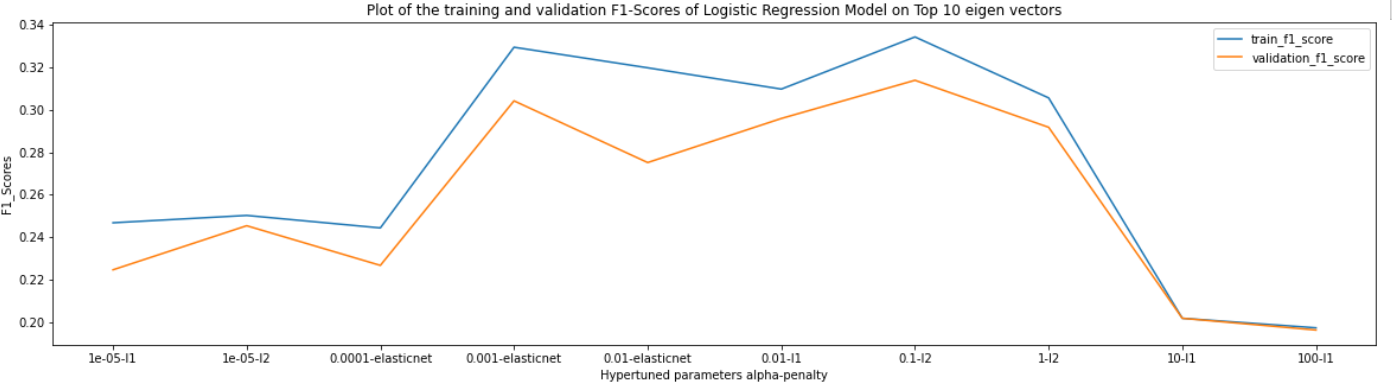Micro Averaged F1-Score plot for various combinations of hyperparameters for Logistic Regression

LINEAR SVM:-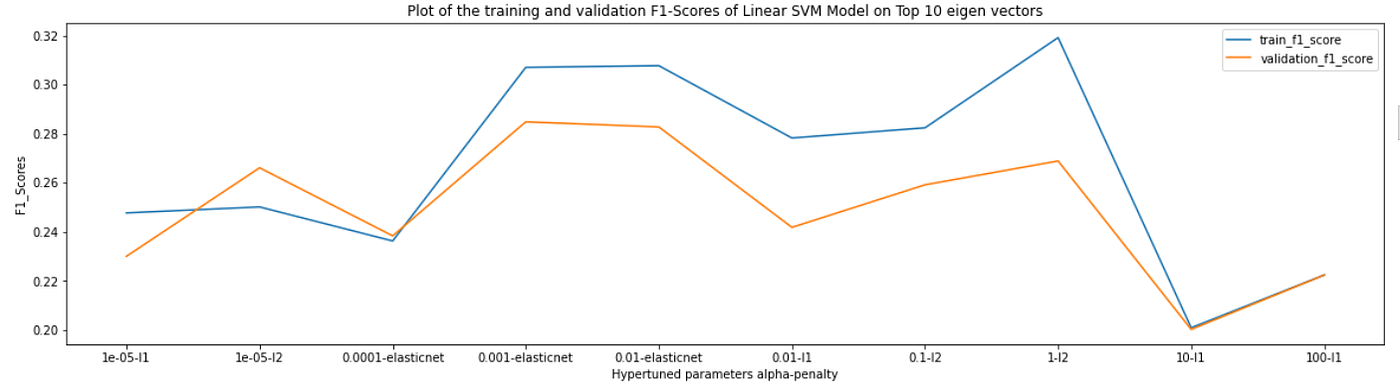Micro Averaged F1-Score Plot for various combinations of hyperparameters for Linear SVM

RANDOM FOREST CLASSIFIER:-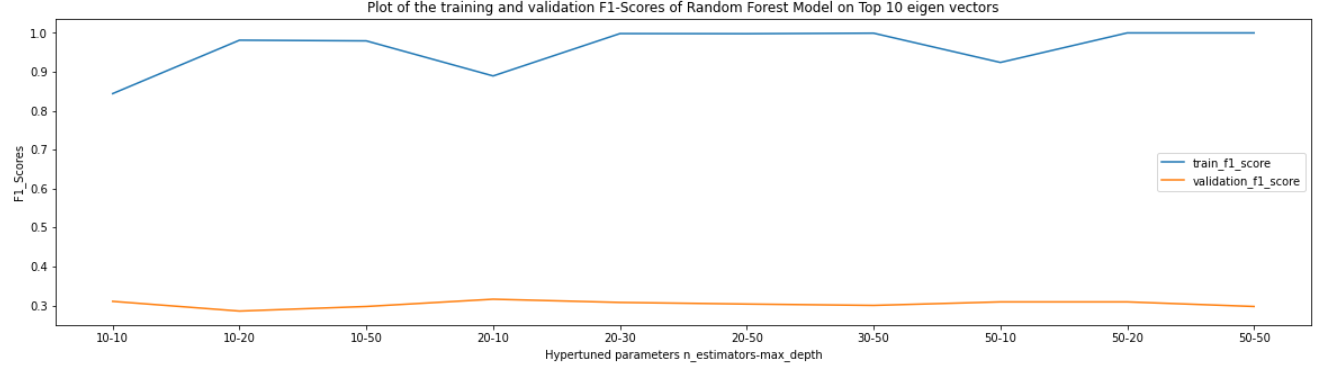Micro Averaged F1-Score Plot for various combinations of hyperparameters for Random Forest Classifier

XGBOOST CLASSIFIER:-

SUMMARY OF VARIOUS ML MODELS:-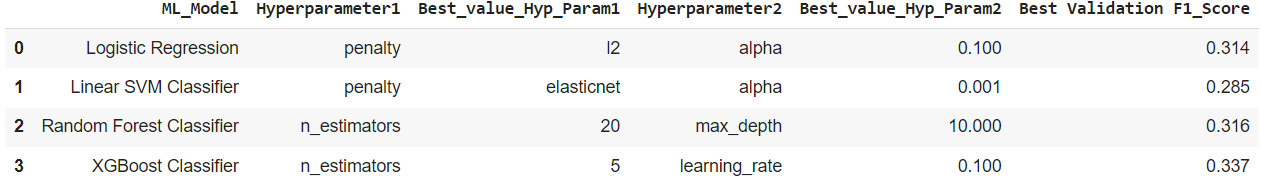Summary of the ML Models trained on the eigen vectors of the visual features

## vii) ML Models on visual features:-

The models Logistic Regression, Linear SVM, Random Forest and XGBoost were applied on the visual features of 64 dimensions and they were validated on the validation data along with hyperparameter tuning done through a randomized search over the hyperparameter space. The thresholds or the best probabilities were chosen so that the micro averaged f1-score can be maximized.

LOGISTIC REGRESSION:-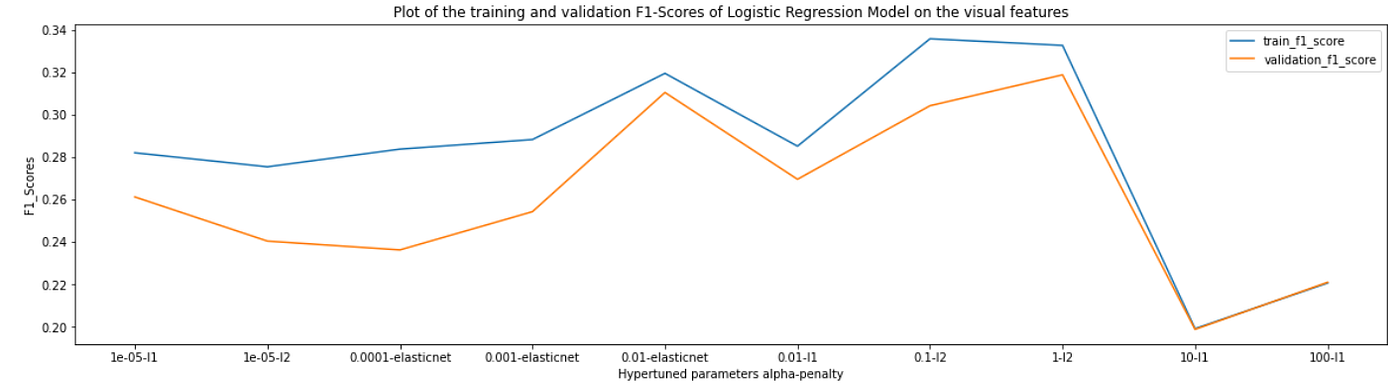Micro Averaged F1-Score Plot for various combinations of hyperparameters for Logistic Regression

LINEAR SVM:-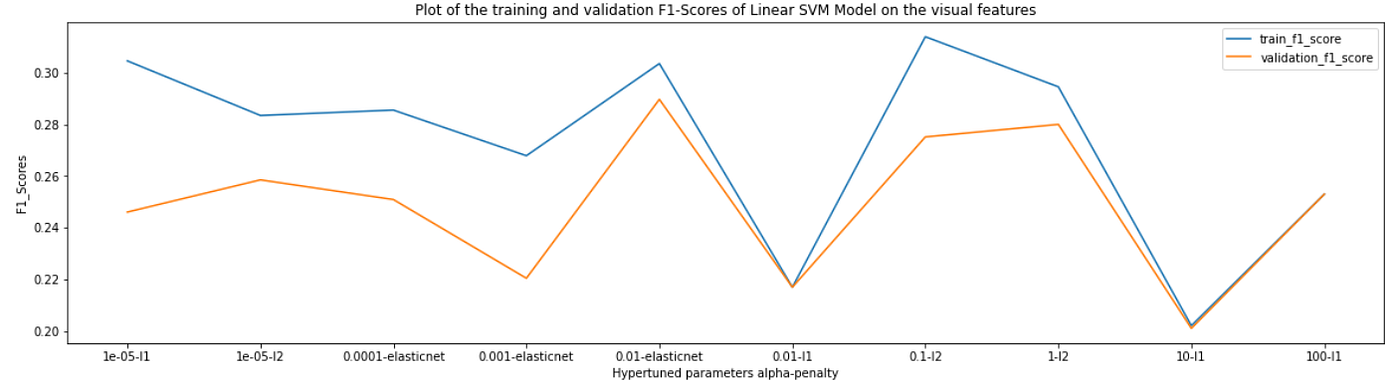Micro Averaged F1-Score Plot for various combinations of hyperparameters for Linear SVM

RANDOM FOREST CLASSIFIER:-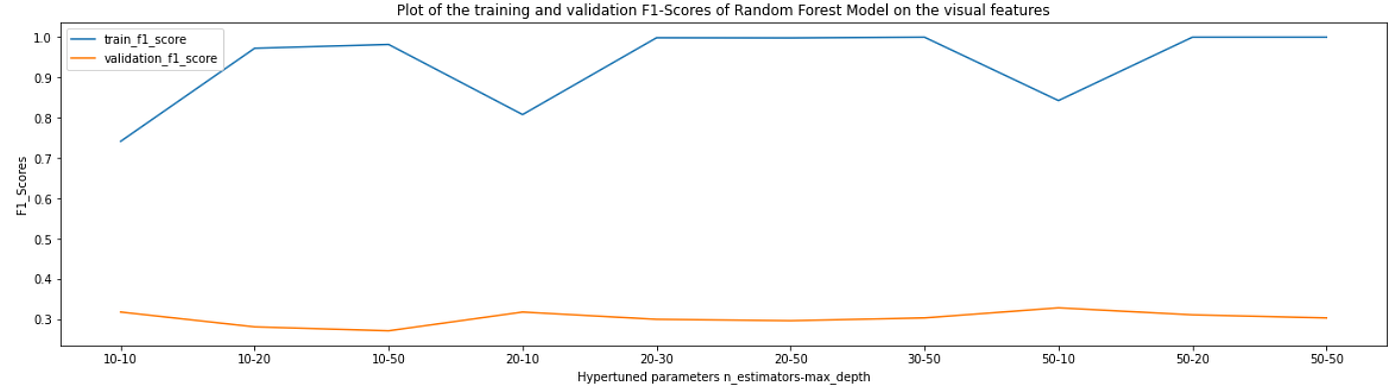Micro Averaged F1-Score Plot for various combinations of hyperparameters for Random Forest Classifier

XGBOOST CLASSIFIER:-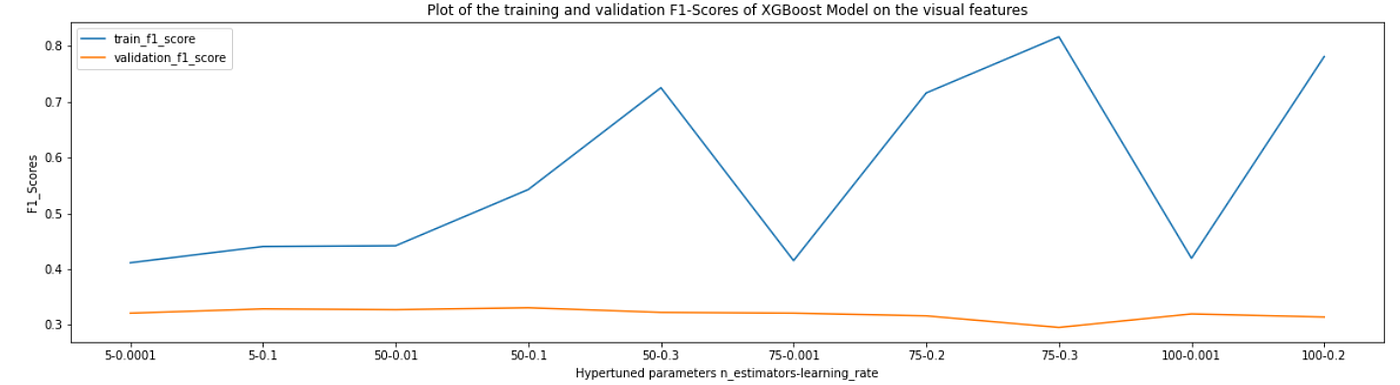Micro Averaged F1-Score Plot for various combinations of hyperparameters for XGBoost Classifier

SUMMARY OF VARIOUS ML MODELS:-

## viii) DL MODEL:-

The final DL Model would take the prediction of the images from the last fully connected layer of the pretrained VGG16 model trained on image categories and then would pass those activations through a dense layer and predict the price range by maximizing f1-score.

ARCHITECTURE:-

In the above model, the input layer itself is coming from the activations of the last full connected layer (FC_2) described in the pretrained model and architecture sub section of this main section.

TENSORBOARD EPOCH LOSS AND MICRO F1_SCORE PLOT:-

The above DL model was trained for 26 epochs with constant learning rate and earlystopping callback. The best micro F1-Score*100 was 35.15.

# CONCLUSION:-

1. All the ML Models and the DL Model performed very poorly on the validation data wrt the micro f1-score.
2. The categorical features age and special discount availability had literally no association with the price ranges of various categories.
3. The VGG16 model did a very good job on separating out the image categories but couldn’t separate out the price range for each of the categories.
4. Each of the price points could be treated as a separate brand for each of the image categories and could have been predicted but that also was a failure as it was tried.
5. Out of the tried models, the DL performed the best achieving 35% micro f1-score which is again very poor.

# FUTURE WORK:-

1. More complex networks like DenseNet and Resnet101 could be tried but there has to be more data for the same.
2. Autoencoders for getting a compressed representation of the images for each category and then training these compressed representation for predicting price range could be tried.

# CODE FOR THE PROJECT:-

Please check the code for the project here:-

# REFERENCES:-

1. https://arxiv.org/abs/1409.1556

--

--

--

## More from Md. Wazir Ali

Love podcasts or audiobooks? Learn on the go with our new app.

## How Big Data Analytics Helps Businesses To Develop New Opportunities## Do Higher Rated Films Really Make More Money? — A Statistical Analysis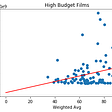## What simple model could we use to forecast Covid-19?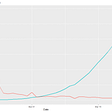## ANOVA, T-test and other statistical tests with Python## Predicting house prices using data driven approach## Data science diary, entry # 1 : Comparing COVID-19 and SARS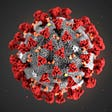## Web Scraping for Data Science — Is it legal?## A Few Musings on Data Quality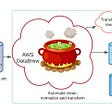## Solidity Value Types Explained## How to get the NFT collection name and URL of an NFT on Solana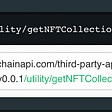## Cloning of “MYNTRA” website